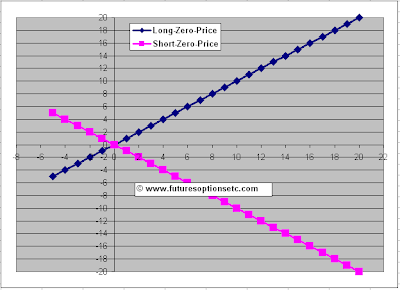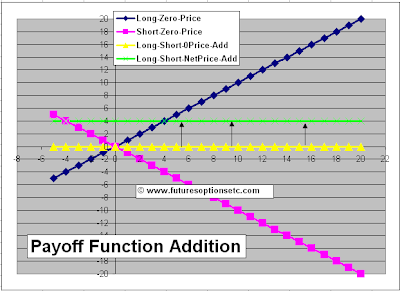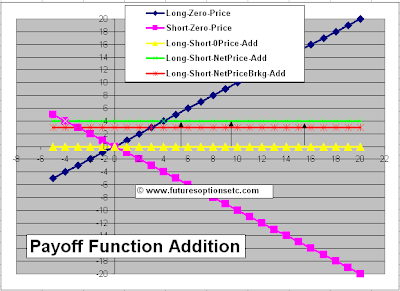The Mathematics of Payoff Functions: Options, Futures, Derivatives & Commodity Trading

# The Mathematics of Payoff Functions

Continuing further from our previous article Payoff Functions for Options & Futures: Explained with Example, in this article we will cover the Mathematics of Payoff Functions.
Before we begin with our example based explanation, please note the following important points:

- Payoff functions can be added and subtracted using simple mathematics (plus and minus)

- Only Payoff functions on the same stock can be added or subtracted. For e.g., 2 or more payoff functions on GOOGLE stock can be added or subtracted, but one payoff function on GOOGLE and another one on MICSOFT can NOT be added or subtracted.

- For Derivatives (options or futures), Payoff functions on the SAME underlying stock or index can be added or subtracted. For e.g. a call option and a put option on the same underlying stock (say Microsoft) can be added or subtracted. But one option on GOOGLE and other on MICROSOFT cannot be added or subtracted

- For Derivatives (options or futures), things become more complex since derivatives also have expiry date. It is possible to add options payoff functions on the same underlying with different expiry dates, but it is a complex procedure. Such cases are typically of calendar spreads, which need to be considered more carefully. See Calendar Spread articles for example.

## Mathematics & Calculations for Payoff Functions

Let's see some examples now:
Example 1)
Suppose you bought 1 share of GOOGLE (Long Position) by paying \$10 on 1 January and after 4 days you Sold 1 share of GOOGLE (short Position) at price of \$14? How do you handle such multiple positions to arrive at the Net payoff functions?
First, draw individual pay off functions for each case. This is displayed by the BLUE colored payoff function for Long (Buy) position, and PINK colored Payoff function for Short (Sell) Position.Now, let's add the two zero priced positions together.
The approach to take while adding the two positions is simple - you first need to identify the regions where the two graphs change their orientation (with respect to each other) or change their values. Sounds difficult, its not.
Just focus on two things for each Payoff function -
(1) the direction and
(2) the magnitude.
Direction can be upward slanting (like Blue Graph) or downward slanting (like Pink graph).
Magnitude is the actual value as per the vertical (y-axis). For e.g., at horizontal point +2, the magnitude value of Blue graph is +2 and the magnitude value of Pink Graph is -2. Add these two and you will get a net value of ZERO.
Similarly, at horizontal point -1, the Blue graph is -1, while the PINK graph is +1. Add these two and you will get a net value of ZERO.
Hence, we need to proceed point by point, adding all these values of the PINK and BLUE colored payoff functions.
Now that may be a difficult and clumsy task, so a better approach is to identify regions. Regions where the graphs change their signs and orientations.
For e.g., If you look at the horizontal region below zero (of horizontal axis), you will observe that in that (below zero) region, the BLUE graph is negative and PINK graph is positive. After the ZERO point (i.e. to the right of zero point), the BLUE Graph becomes positive and PINK graph becomes negative. Hence, we have 2 regions to consider.
i - Below ZERO - The blue graph is slanting upwards and is negative. The Pink graph is slanting downwards and is positive. If you add the two, you will get a ZERO value.
ii - Above Zero - The blue graph is slanting upwards and is positive. The Pink graph is slanting downwards and is negative. If you add the two, you will get a ZERO value.

Hence, in both the regions, the net sum of the two payoff functions comes to zero. Hence, this simple case of one long and one short payoff functions gives you a NET zero payoff function. That is now indicated by a YELLOW colored NET Payoff function, which is nothing by a ZERO horizontal line.Still not understood, don't worry. Just keep reading through the rest of the examples below and it will become clear.

Now, let's adjust the net payoff function for the net price.
First what is the net price of these one long and one short payoff functions? The Buy position costs you \$10 and the Sell position gives you \$14. Hence, you receive a NET (\$14 - \$10 = \$4). Since this is a net inflow of \$4, we need to shift the net payoff function graph (YELLOW payoff function) UPWARDS by \$4.Once we do it, we get the GREEN colored payoff function, which represents the real net profit and loss from this Long and Short position. And this is the step-by-step method to add the payoff functions. (Read more examples below for more understanding).
To verify, we bought (Long) the stock at \$10 and sold (Short) at \$14. Net profit to us is \$4 and that's what is reflected correctly by the final GREEN colored payoff function.
But note, although we have considered the net price, we have still NOT considered the brokerage. Assume that we pay \$0.5 brokerage on Buy side, and another \$0.5 on Sale side position, total outflow for brokerage is \$1. Since this is a cost (net outflow) we need to shift the GREEN payoff function downwards by \$1 to get the true payoff function (RED payoff function as follows):May be this example being the first one might be difficult to understand. Let's see some more examples of Payoff functions for better understanding. Head on to the next set of Payoff Function Examples for Options
 Posted by Shobhit See All Articles with